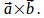## Proof That For an Ellipse, the Dot Product of the Curve With the Cross Product of the Tangent Vector With the Second Derivative is Zero

Theorem

For a curve defined by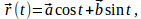where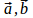are constant vectors,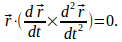Theorem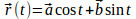so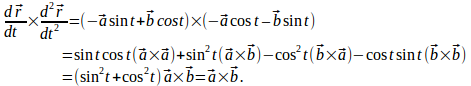Hence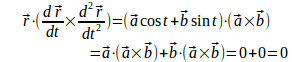since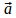and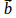are perpendicular to